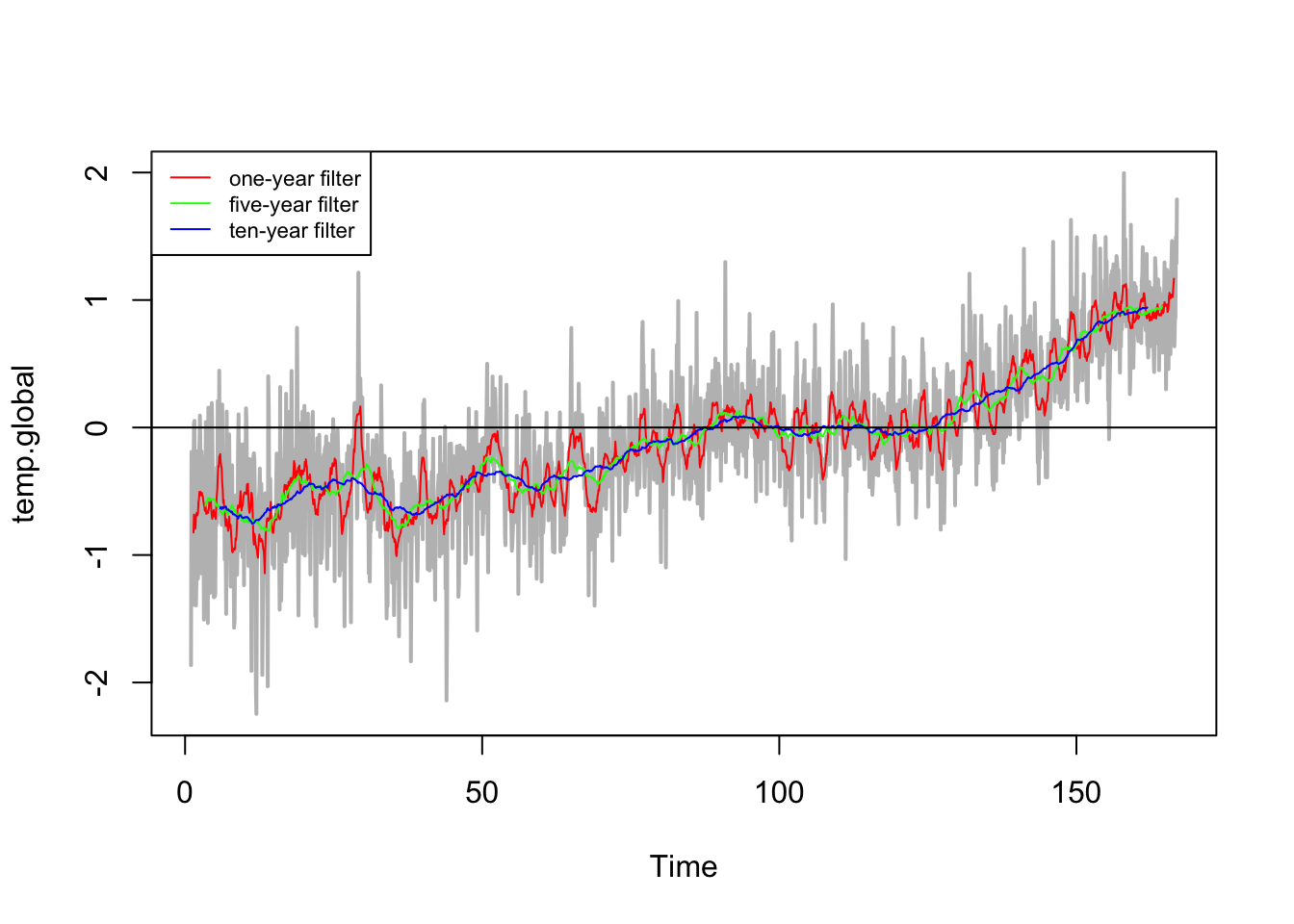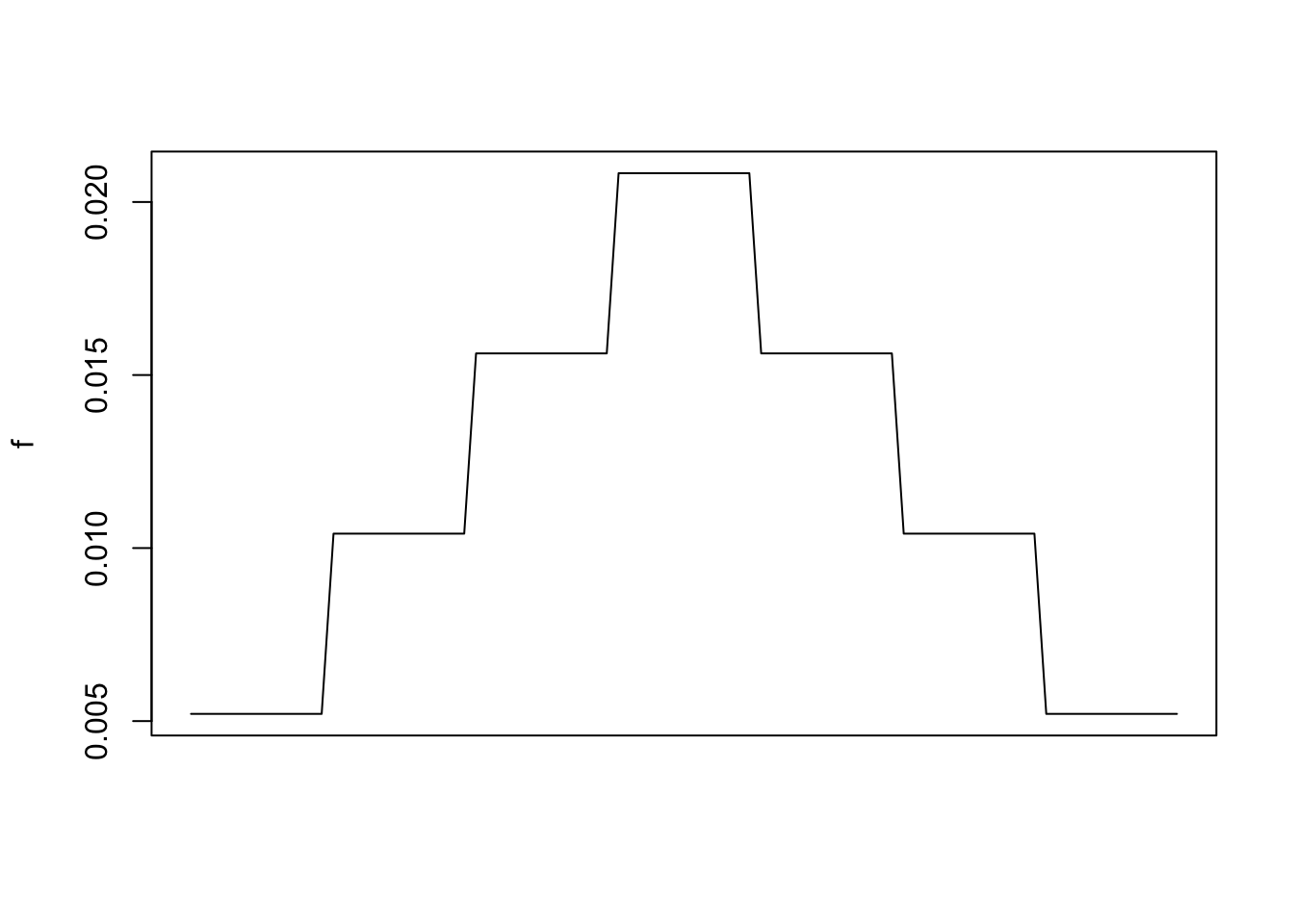A symmetric moving average $$(m_t)$$ for the observation $$x_t$$ is given by

$m_t = \sum_{j=-k}^k a_jx_{t-j}\text{,}$

where $$a_j = a_{-j}$$ and $$\sum_{j=-k}^ka_j = 1$$.

A simple way to apply a moving average smoother (low-pass filter) in R is to apply a linear filter, using the filter() function. By default the method uses a moving average of values on both sides (before and after) each measurement. To specify the nature of our low-pass filter we have to specify a vector of filter coefficients, which we provide to the filter argument in the filter() function.

Consider a monthly time series. In order to construct a symmetric 3-month low pass filter we construct a vector of that form:

rep(1, 3)/3
##  0.3333333 0.3333333 0.3333333

This low pass filter can be written in mathematical notation as

$m_t = \frac{1}{3}(x_{t-1}+x_t+x_{t+1})$

In contrast a symmetric one-year filter is specified by

rep(1, 12)/(12)
##   0.08333333 0.08333333 0.08333333 0.08333333 0.08333333 0.08333333
##   0.08333333 0.08333333 0.08333333 0.08333333 0.08333333 0.08333333

Let us build three low pass filters for a the global earth surface temperature anomalies data set:

• a symmetric one-year filter
• a symmetric 5-year filter
• a symmetric 10-year filter

As the function name filter is a quite common word, we explicitly state that we refer to the filter() function of the stats package by typing stats::filter().

library(xts)

temp.global.f1 <- stats::filter(temp.global,
filter = rep(1, 12)/12)
temp.global.f5 <- stats::filter(temp.global,
filter = rep(1, 12*5)/(12*5))
temp.global.f10 <- stats::filter(temp.global,
filter = rep(1, 12*10)/(12*10))

## plotting ##
plot.ts(temp.global, lwd = 2, col = 'gray')
lines(temp.global.f1, col = 'red')
lines(temp.global.f5, col = "green")
lines(temp.global.f10, col = "blue")
abline(h = 0)
legend('topleft', legend = c('one-year filter',
'five-year filter',
'ten-year filter'),
col = c('red','green','blue'),
lty = 1, cex = 0.7)The filter() function is quite flexible, hence it is straightforward to define a custom filter. Let us filter the time series with a symmetric seven-year filter that gives full weight to the measurement year, three-quarters weight to adjacent year, half weight to years two remoted, and quarter weight to years three remoted.

f <- c(0.25, 0.5, 0.75, 1, 0.75, 0.5, 0.25) # construct pattern
f <- rep(f, each = 12) # account for monthly data structure of the time series
f <- f/sum(f) # weights
plot(f, type = 'l', xlab = "", xaxt = "n") # plot for a better understandingLet us apply our custom symmetric seven-year filter and plot the result.

temp.global.f <- stats::filter(temp.global, filter = f)
plot.ts(temp.global, lwd = 2, col = 'gray')
lines(temp.global.f, col = "red")
legend('topleft',
legend = c('monthly data',
'custom seven-year filter'),
col = c('gray', 'red'),
lty = 1,
lwd = c(2,1),
cex = 0.7)
abline(h = 0)